# Getting to the Bottom of Matrix Completion and Nonnegative Least Squares with the MM Algorithm

## Features

• Author: Jocelyn T. Chi and Eric C. Chi
• Date: 31 Mar 2014
• Copyright: Image appears courtesy of iStock Photo

## Introduction

The Expectation Maximization (EM) algorithm has a rich and celebrated role in computational statistics. A hallmark of the EM algorithm is its numerical stability. Because the iterates of the EM algorithm always make steady monotonic progress towards a solution, iterates never wildly overshoot the target. The principles that lead to this monotonic behavior, however, are not limited to estimation in the context of missing data. In this article, we review its conceptually simpler generalization, the majorization-minimization (MM) algorithm, that embodies the core principles that give rise to the numerical stability enjoyed by the EM algorithm.1 We will see that despite its simplicity, the basic MM strategy can take us surprisingly far. MM algorithms are applicable to a broad spectrum of non-trivial problems and often yield algorithms that are almost trivial to implement.

The basic idea behind the MM algorithm is to convert a hard optimization problem (for example, non-differentiable, non-convex, or constrained) into a sequence of simpler ones (for example, smooth, convex, or unconstrained). Thus, like the EM algorithm, the MM algorithm is not an algorithm, but a principled framework for the construction of one. As a preview of things to come, we will describe the MM algorithm framework and show how the gradient descent algorithm we discussed in the first article is an instance of the MM algorithm. This will give the intuitive picture of how the MM algorithm works. We then dive right into two case studies in the application of MM algorithms to the matrix completion and nonnegative least squares problems. We discuss implementation considerations along the way.## Overview of the MM Algorithm

Consider the basic optimization problem

\begin{align} &\text{minimize } f({\bf x}) \\ &\text{subject to } {\bf x} \in \mathbb{R}^{n}. \end{align}

Sometimes, it may be difficult to optimize $$f({\bf x})$$ directly. In these cases, the MM framework can provide a solution to minimizing $$f({\bf x})$$ through the minimization of a series of simpler functions. The resulting algorithm alternates between taking two kinds of steps: majorizations and minimizations. The majorizing step identifies a suitable surrogate function for the objective function $$f({\bf x})$$, and the minimizing step identifies the next iterate through minimization of the surrogate function. In the following section, we discuss these two steps in greater detail.

### The Two Steps of the MM Algorithm

Suppose we have generated $$n$$ iterates, the $$n^{\text{th}}$$ of which we denote by $${\bf x}_{n}$$. We describe how to generate the $$n+1^{\text{th}}$$ iterate. The first step of the MM algorithm framework is to majorize the objective function $$f({\bf x})$$ with a surrogate function $$g({\bf x} | {\bf x}_{n})$$ anchored at $${\bf x}_{n}$$. The majorizing function $$g({\bf x} | {\bf x}_{n})$$ must satisfy two conditions.

1. The majorizing function must equal the objective function at the anchor point. This tangency condition requires that $$g({\bf x}_{n} | {\bf x}_{n}) = f({\bf x}_{n})$$.

2. The majorizing function must be at least as great as the objective function at all points. This domination condition requires that $$g({\bf x} | {\bf x}_{n}) \ge f({\bf x})$$ for all $${\bf x}$$.

Thus, a majorization touches the objective function at the anchor point and lies above it everywhere else.

Once a suitable surrogate function has been found, the second step of the MM algorithm framework is to minimize the surrogate function $$g({\bf x} | {\bf x}_{n})$$. Let $${\bf x}_{n+1}$$ denote a minimizer of $$g({\bf x} | {\bf x}_{n})$$. Then the tangency and domination conditions guarantee that minimizing the majorization results in a descent algorithm since

\begin{align} f({\bf x}_{n+1}) \le g({\bf x}_{n+1} | {\bf x}_{n}) \le g({\bf x}_{n} | {\bf x}_{n}) = f({\bf x}_{n}). \end{align}

Consider these comparisons from left to right. The first inequality is due to the domination condition, the second arises since $${\bf x}_{n+1}$$ is the minumum of $$g({\bf x} | {\bf x}_{n})$$, and the third is a result of the tangency condition. Hence, we are assured that the algorithm produces iterates that make monotonic progress towards minimizing the objective function. We repeat these two steps using the new minimizer $${\bf x}_{n+1}$$ as the anchor for the subsequent majorizing function. In practice, exact minimization of the surrogate function is not essential since the domination and tangency conditions guarantee the descent property of the algorithm.

The “MM” algorithm derives its name from the two-step majorization-minimization process for minimization problems, or minorization-maximization for maximization problems. The idea is that it should be much easier to minimize the surrogate function than it would be to minimize the original objective function. The art, of course, is in finding a surrogate function whose minimizer can be simply derived explicitly, or determined quickly with iterative methods.

## Revisiting Gradient Descent as an MM Algorithm

In the gradient descent tutorial, we saw how different step-size values $$\alpha$$ in the gradient descent algorithm lead to different quadratic approximations for the objective function, shown below in blue.

library(gettingtothebottom) 
## Loading required package: ggplot2  ## Loading required package: grid  ## Loading required package: Matrix 
example.quadratic.approx(alpha1 = 0.01, alpha2 = 0.12)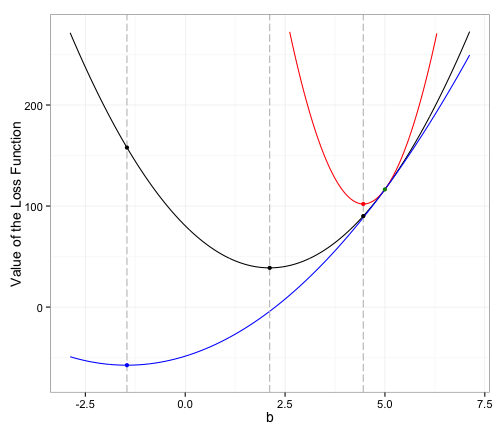In the figure above, the black and red quadratic approximations determined by the different step-sizes also result in two majorizing functions. Both the black and red curves are tangent to the blue curve at the anchor point, depicted by the green dot. The curves also dominate the blue curve at all values of $${\bf b}$$.

Quadratic majorizations are particularly powerful and useful. In the next section, we discuss how a simple quadratic majorizer can help us solve matrix completion problems.

## Matrix Completion

Between October 2006 and September 2009, Netflix, an American on-demand online streaming media provider, hosted the Netflix Prize challenge, a competition for the best algorithm to predict movie preferences based on user movie ratings. The competition featured a 1 million grand prize and a large training dataset containing approximately $$480,000$$ user ratings on $$18,000$$ movies with over $$98$$ percent missing data. Movie Titles Anna Ben Carl Star Wars 2 5 ? Harry Potter ? 1 ? Miss Congeniality 1 5 1 Lord of the Rings 5 2 ? The matrix above depicts how a miniscule portion of the training dataset may have appeared. In this example, Anna, Ben, and Carl have each rated a small number movies. The challenge would be to come up with an algorithm to predict what kind of movie Anna would want to watch based on her own ratings, and ratings by Netflix users with similar movie preferences. ### Representation as the Matrix Completion Problem Predicting unobserved movie ratings is an example of the matrix completion problem. In a matrix completion problem, we seek to “complete” a matrix of partially observed entries with the “simplest” complete matrix consistent with the observed entries in the original data. In this case, we take simplicity to mean low rank. We say that a matrix is rank-$$r$$ if it can be expressed as the weighted sum of $$r$$ rank-$$1$$ matrices, ${\bf X} = \sum_{i=1}^{r} \sigma_{i}{\bf u}_{i}{\bf v}_{i}^{t}.$ Every matrix $${\bf X}$$ admits a decomposition of the above form, called the singular value decomposition (SVD). The decomposition can be rewritten as $${\bf X} = {\bf U}{\bf D}{\bf V}^{t}$$, where the columns of $${\bf U}$$ and $${\bf V}$$ are orthonormal (i.e. $${\bf U}^{t}{\bf U} = {\bf I}$$ and $${\bf V}^{t}{\bf V} = {\bf I}$$), and $${\bf D}$$ is a diagonal matrix containing the ordered singular values of $${\bf X}$$ such that ${\bf D} = \begin{pmatrix}\sigma_{1} & & 0 \\ & \ddots & \\ 0 & & \sigma_{r} \end{pmatrix},$ with $$\sigma_{1} \ge \sigma_{2} \ge \dots \ge \sigma_{r} > 0$$. Since we seek the model matrix $${\bf Z}$$ that is most consistent with the original data matrix $${\bf X}$$, we require a measure of closeness between the data and the model. To do that, we can employ the Frobenius norm of a matrix, given by $\lVert {\bf X} \rVert_{\text{F}} = \sqrt{\sum_{i=1}^{r}\sigma_{i}^{2}}.$ The matrix completion problem can then be stated as the following optimization problem $\text{minimize}\; \frac{1}{2} \lVert P_{\Omega^{c}}({\bf X}) - P_{\Omega^{c}}({\bf Z}) \rVert_{\text{F}}^{2},$ subject to the constraint that rank$$({\bf Z}) \leq r$$, where $${\bf X}$$ is the original data, $${\bf Z}$$ is the model, and $$\Omega$$ denotes the set of unobserved indices. Note that $$r$$ is a user-defined upper limit to the rank of the matrix or complexity of the model. The function $$P_{\Omega^{c}}({\bf Z})$$ is the projection of the matrix $${\bf Z}$$ onto the set of matrices with zero entries given by indices in the set $$\Omega$$, namely \begin{align} [P_{\Omega^c}({\bf Z})]_{ij} = \begin{cases} z_{ij} & \text{ for (i,j) \not\in \Omega, and} \\ 0 & \text{ otherwise.} \end{cases} \end{align} The $$\frac{1}{2} \lVert P_{\Omega^{c}}({\bf X}) - P_{\Omega^{c}}({\bf Z}) \rVert_{\text{F}}^{2}$$ portion of the objective penalizes differences between $${\bf X}$$ and $${\bf Z}$$ over the observed entries. This formulation balances the tradeoff between the complexity (rank) of the model and how well the model matches the data. As we relax the bound on the rank $$r$$, we can better fit the data at the risk of overfitting it. Unfortunately, the formulated problem is difficult to solve, since the rank constraint makes the optimization task combinatorial in nature. Using matrix rank as a notion of complexity, however, is a very useful idea in many applications. In the Netflix data, the low rank assumption is one way to model our belief that there are a relatively small number of fundamental movie genres and moviegoer tastes that can explain much of the systematic variation in the ratings. We can reach a compromise and relax the problem by substituting an easier objective to minimize that still trades off the goodness of fit with the matrix rank. In place of a rank constraint, we tack on a regularization term, or penalty, on the nuclear (or trace) norm of the model matrix $${\bf Z}$$. The nuclear norm is given by $\lVert {\bf X} \rVert_{\ast} = \sum_{i=1}^{r} \sigma_{i},$ and it is known to be a good surrogate of the rank in matrix approximation problems. Employing it as a regularizer results in the following formulation $\text{minimize } \frac{1}{2} \lVert P_{\Omega^{c}}({\bf X}) - P_{\Omega^{c}}({\bf Z}) \rVert_{\text{F}}^{2} + \lambda \lVert {\bf Z} \rVert_{\ast},$ where the tuning parameter $$\lambda \geq 0$$ trades off the complexity of the model with how well the model agrees with the data over the observed entries. Although we have made progress towards solving a simpler problem, the presence of the projection operator $$P_{\Omega^{c}}$$ still presents a challenge. This is where majorization comes to our aid. We next derive a quadratic majorization of the troublesome squared error term in our objective. First note that we can rewrite this term more verbosely as \begin{align} \frac{1}{2} \lVert P_{\Omega^{c}}({\bf X}) - P_{\Omega^{c}}({\bf Z}) \rVert_{\text{F}}^{2} &= \frac{1}{2} \sum_{(i,j) \in \Omega^{c}}(x_{ij} - z_{ij})^{2}. \end{align} Suppose we already have the $$n^{\text{th}}$$ iterate $${\bf Z}^{(n)}$$. Observe that the following quadratic function of $${\bf Z}$$ is always nonnegative \begin{align} 0 \leq \frac{1}{2} \sum_{(i,j) \in \Omega} (z_{ij} - z_{ij}^{(n)})^{2}, \\ \end{align} and the inequality becomes equality when $${\bf Z} = {\bf Z}^{(n)}$$. Adding the above inequality to the equality above it produces the desired quadratic majorization anchored at the $$n^{\text{th}}$$ iterate $${\bf Z}^{(n)}$$ \begin{align} \frac{1}{2} \lVert P_{\Omega^{c}}({\bf X}) - P_{\Omega^{c}}({\bf Z}) \rVert_{\text{F}}^{2} &\leq \frac{1}{2} \sum_{(i,j) \in \Omega^{c}}(x_{ij} - z_{ij})^{2} + \frac{1}{2} \sum_{(i,j) \in \Omega} (z_{ij} - z_{ij}^{(n)})^{2} \\ &= \frac{1}{2} \sum_{(i,j)}(y_{ij}-z_{ij})^{2} \\ &= \frac{1}{2} \lVert {\bf Y} - {\bf Z} \rVert ^{2}_{\text{F}}, \end{align} where \begin{align} y_{ij} =\begin{cases} x_{ij} & \text{for (i,j) \not\in \Omega,} \\ z_{ij}^{(n)} & \text{for (i,j) \in \Omega}. \end{cases} \end{align} Thus, we have converted our original problem to one of repeatedly solving the following problem \begin{align} \text{minimize } \frac{1}{2}\lVert {\bf Y} - {\bf Z} \rVert^{2}_{\text{F}} + \lambda \lVert {\bf Z} \rVert_{\ast}. \end{align} Taking a step back, we see that the MM principle has allowed us to replace a problem with missing entries with one without missing entries. This majorization is very straighforward to minimize compared to the original problem. In fact, minimizing the majorization can be thought of as a matrix version of the ubiquitous lasso problem and can be accomplished in four steps. One of those steps involves the soft-threshold operator, synonymous with the lasso, which is itself the solution to an optimization problem involving the 1-norm and is given by \begin{align} S(s,\lambda) &= \text{arg min } \frac{1}{2}(s-t)^{2} + \lambda \lvert t \rvert \\ &= \begin{cases} s-\lambda & \text{if s \ge \lambda},\\ s + \lambda & \text{if s \le -\lambda}, \text{ and}\\ 0 & \text{\lvert s \rvert < \lambda }. \end{cases} \end{align} The figure below illustrates what the soft-threshold operator does. The gray line depicts the line $$f(x) = x$$ and the blue curve indicates how the soft-threshold function $$S(x,\lambda)$$ shrinks $$f(x)$$ by sending $$f(x) \in (-\lambda, \lambda$$) to zero, and shrinking all other $$f(x)$$ towards zero by a constant amount $$\lambda$$. Note: If you already installed the gettingtothebottom package for the gradient descient tutorial, you'll need to update the package to version 2.0 in order to call the following functions. If you are using RStudio, you can do that by selecting Tools > Check for Package Updates. plot_softhreshold(from = -5, to = 5, lambda = 3)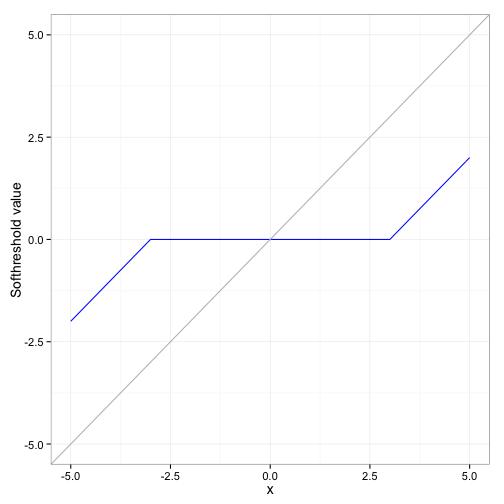The minimizer to the majorization is given by $${\bf Z}={\bf U}S({\bf D},\lambda){\bf V}^{t}$$, where $${\bf Y}$$ has the singular value decomposition $${\bf U}{\bf D}{\bf V}^{t}$$ and $$S({\bf D},\lambda)$$ denotes the application of the soft-threshold operator on the elements of the matrix $${\bf D}$$, namely \begin{align} S({\bf D},\lambda) = \begin{pmatrix} S(\sigma_{1},\lambda) & & 0 \\ & \ddots & \\ 0 & & S(\sigma_{r},\lambda) \end{pmatrix}. \end{align} We provide a sketch of how we derived the minimizer of the majorization later in this article. ### MM Algorithm for Matrix Completion A formalization of the steps described above presents the following MM algorithm for matrix completion. Given a data matrix $${\bf X}$$, a set of unobserved indices $$\Omega$$, and an initial model matrix $${\bf Z}^{(0)}$$, repeat the following four steps until convergence. \begin{align} & \text{1. } y^{(n+1)}_{ij} = \begin{cases} x_{ij} & \text{for (i,j) \not\in \Omega}, \text{ and}\\ z_{ij}^{(n)} & \text{for (i,j) \in \Omega}. \end{cases} \\ & \text{2. } ({\bf U},{\bf D},{\bf V}) = \text{SVD}[{\bf Y}^{(n+1)}] \\ & \text{3. } \tilde{{\bf D}} = S({\bf D},\lambda) \\ & \text{4. } {\bf Z}^{(n+1)} = {\bf U}\tilde{{\bf D}}{\bf V}^{t} \end{align} This imputation method is known as the soft-impute algorithm. In words, we fill in the missing values using the relevant entries from our most recent MM iterate to get $${\bf Y}$$. We then take $${\bf Y}$$ apart by factoring it into the matrices $${\bf U}, {\bf D},$$ and $${\bf V}$$ with an SVD and soft-threshold the diagonal matrix $${\bf D}$$ to obtain a new diagonal matrix $$\tilde{\bf D}$$. Finally, to get the next iterate we put the pieces back together, substituing $$\tilde{\bf D}$$ for $${\bf D}$$. Since both the majorization and objective functions are convex, the algorithm is guaranteed to proceed towards a global minimum. Hence, the choice of the initial guess for the model matrix $${\bf Z}$$ is not materially important for eventually reaching a solution, although an informed choice is likely get to a good answer sooner. We will give some guidance on how to make that informed choice in a moment. Convergence may be defined by several metrics. As the algorithm approaches convergence, the change in the iterates (and the resulting objective function values) will be minimal. Hence, we may decide to terminate the MM algorithm once the absolute difference in the objective function values of adjacent iterates falls below some small threshold. Alternatively, the relative change in adjacent iterates may also be used. In the matrixcomplete function of the gettingtothebottom package, we watch the relative change in the iterates to decide when to halt our algorithm. We further notice that this algorithm provides a solution $${\bf Z}$$ for a given value of $$\lambda$$. In practice, the algorithm is typically run over a sequence of decreasing $$\lambda$$ values. The resulting sequence of solutions is called the regularization, or solution, path. This path of solutions may then be used to identify the $$\lambda$$ value that minimizes the error between the data $${\bf X}$$ and the model $${\bf Z}$$ on a held-out set of entries. We do not go into detail on how to choose $$\lambda$$ in this article. Instead, since we illustrate the method on simulated data, we are content to show that there is a $$\lambda$$ which minimizes the true prediction error on the unobserved entries. Before moving on to some R code, we point out that using the solution at the previous value of $$\lambda$$ as the initial value $${\bf Z}^{(0)}$$ for the next lower value of $$\lambda$$ in the sequence of $$\lambda$$'s can drastically reduce the number of iterations. This practice is often referred to as using “warm starts” and leverages the fact that solutions to problems with similar $$\lambda$$'s are often close to each other. ### An MM Algorithm for Matrix Completion The following example implements the MM algorithm for matrix completion described above. In our code, we store the set of missing indices $$\Omega$$ as a vector. # Generate a test matrix set.seed(12345) m <- 1000 n <- 1000 r <- 5 Mat <- testmatrix(m, n, r) # Add some noise to the test matrix E <- 0.1 * matrix(rnorm(m * n), m, n) A <- Mat + E # Obtain a vector of unobserved entries temp <- makeOmega(m, n, percent = 0.5) omega <- tempomega   # Remove unobserved entries from test matrix  X <- A  X[omega] <- NA   # Make initial model matrix Z and find initial lambda  Z <- matrix(0, m, n)  lambda <- init.lambda(X, omega)   # Run example  Sol <- matrixcomplete(X, Z, omega, lambda) 
## Optimization completed. 
# Error (normed difference)  diff_norm(Sol$sol, A, omega)  ##  121.5  In this example, we utilized the init.lambda function to obtain an initial value for $$\lambda$$. For a sufficiently large $$\lambda$$, the solution is the matrix of all zeros, namely $${\bf Z} = {\bf 0}$$. The smallest $$\lambda$$ that gives this answer is given by the largest singular value of a matrix that equals $${\bf X}$$ on $$\Omega^c$$ and is zero on $$\Omega$$. The init.lambda function returns this value. We use this $$\lambda$$ as the first of a decreasing sequence of regularization parameters, since we can make an informed choice on the first initial starting point, namely $${\bf Z}^{(0)} = {\bf 0}$$. If the next smaller $$\lambda$$ is not too far from the $$\lambda$$ returned by init.lambda our solution to the first problem, namely $${\bf Z} = 0$$, should not be too far from the solution to the current problem. As we progress through our sequence of decreasing $$\lambda$$ values, we repeatedly recycle the solution at one $$\lambda$$ as the initial guess $${\bf Z}^{(0)}$$ for the next smaller $$\lambda$$. # Generate a test matrix seed <- 12345 m <- 100 n <- 100 r <- 3 Mat <- testmatrix(m, n, r, seed = seed) # Add some noise to the test matrix E <- 0.1 * matrix(rnorm(m * n), m, n) A <- Mat + E # Obtain a vector of unobserved entries temp <- makeOmega(m, n, percent = 0.5) omega <- temp$omega   # Remove unobserved entries from test matrix  X <- A  X[omega] <- NA   # Make initial model matrix Z and find initial lambda  Z <- matrix(0, m, n)  lambda.start <- init.lambda(X, omega)  lambdaseq_length = 20  tol <- 0.01   ans <- solutionpaths(A, X, Z, omega, lambda.start, tol = tol, liveupdates = TRUE, lambdaseq_length = lambdaseq_length) 
## Optimization completed.  ## Completed results for lambda  1  of  20 .  ## Completed iteration  1 .  ## Completed iteration  2 .  ## Completed iteration  3 .  ## Completed iteration  4 .  ## Completed iteration  5 .  ## Optimization completed.  ## Completed results for lambda  2  of  20 .  ## Completed iteration  1 .  ## Completed iteration  2 .  ## Completed iteration  3 .  ## Completed iteration  4 .  ## Optimization completed.  ## Completed results for lambda  3  of  20 .  ## Completed iteration  1 .  ## Completed iteration  2 .  ## Completed iteration  3 .  ## Completed iteration  4 .  ## Optimization completed.  ## Completed results for lambda  4  of  20 .  ## Completed iteration  1 .  ## Completed iteration  2 .  ## Completed iteration  3 .  ## Optimization completed.  ## Completed results for lambda  5  of  20 .  ## Completed iteration  1 .  ## Completed iteration  2 .  ## Completed iteration  3 .  ## Optimization completed.  ## Completed results for lambda  6  of  20 .  ## Completed iteration  1 .  ## Completed iteration  2 .  ## Completed iteration  3 .  ## Optimization completed.  ## Completed results for lambda  7  of  20 .  ## Completed iteration  1 .  ## Completed iteration  2 .  ## Optimization completed.  ## Completed results for lambda  8  of  20 .  ## Completed iteration  1 .  ## Completed iteration  2 .  ## Optimization completed.  ## Completed results for lambda  9  of  20 .  ## Completed iteration  1 .  ## Optimization completed.  ## Completed results for lambda  10  of  20 .  ## Completed iteration  1 .  ## Optimization completed.  ## Completed results for lambda  11  of  20 .  ## Completed iteration  1 .  ## Optimization completed.  ## Completed results for lambda  12  of  20 .  ## Completed iteration  1 .  ## Optimization completed.  ## Completed results for lambda  13  of  20 .  ## Completed iteration  1 .  ## Optimization completed.  ## Completed results for lambda  14  of  20 .  ## Optimization completed.  ## Completed results for lambda  15  of  20 .  ## Optimization completed.  ## Completed results for lambda  16  of  20 .  ## Optimization completed.  ## Completed results for lambda  17  of  20 .  ## Optimization completed.  ## Completed results for lambda  18  of  20 .  ## Optimization completed.  ## Completed results for lambda  19  of  20 .  ## Optimization completed.  ## Completed results for lambda  20  of  20 . 

The following figure is a plot of the true values (obtained from the data we generated in $${\bf A}$$) against the imputed values in the minimum error solution found using our comparison in the solutionpaths function. The plot shows the errors using the model with the $$\lambda$$ that gives the solution with the least discrepancy with $${\bf A}$$. Of course, in practice we do not know $${\bf A}$$. The point we want to make is that there is a $$\lambda$$ that can lead to good predictions. The plot indicates that the matrix completion algorithm does well in finding a complete model $${\bf Z}$$ that is similar to $${\bf X}$$ since the imputed values are quite similar to the true values in our example. You will notice, however, that the imputed entries are all biased towards zero. This is an artifact of using the nuclear norm heuristic in place of the rank constraint. It is a small price to pay for a computationally tractable model. This bias is in fact a common theme in penalized estimation. Penalties like the 1-norm in the lasso and 2-norm in ridge regression, trade off increased bias for reduced variance to achieve an overall lower mean squared error.

plot_solpaths_error(A, omega, ans)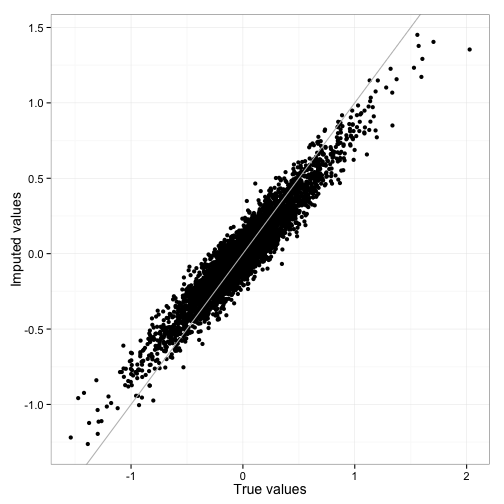The figure below depicts the result of the comparisons from the solutionpaths function. The figure shows a plot of the error from each $$\lambda$$ in our comparison as a function of $$\log_{10}(\lambda)$$ (with rounded $$\lambda$$ values below each point). The blue line indicates the $$\lambda$$ value resulting in the minimum normed difference error between the data and the solution model. We observe that higher $$\lambda$$ values resulted in larger errors, and as $$\lambda$$ moved towards zero, the change in the model error also decreases. Although it is hard to see on the graph, the error actually increased again after some $$\lambda$$ value.

plot_solutionpaths(ans)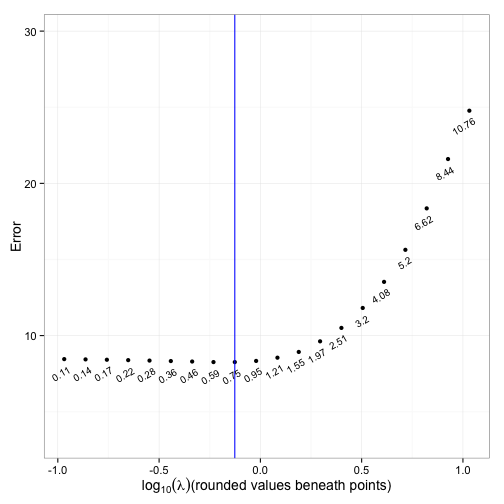We conclude our brief foray into matrix completion with an abridged derivation of the minimizer of the majorizing function.

### Derivation of the minimizer of the majorization

In deriving the MM algorithm we used the fact that the following function $\begin{eqnarray} \frac{1}{2} \lVert {\bf Y} - {\bf Z} \rVert_{\text{F}}^2 + \lambda \lVert {\bf Z} \rVert_\ast \end{eqnarray}$ has a unique global minimizer $${\bf Z}^\star = {\bf U}{\bf D}{\bf V}^{t}$$, where $${\bf Y} = {\bf U}{\bf \Sigma}{\bf V}^{t}$$ is the singular value decomposition of $${\bf Y}$$ and $${\bf D}$$ is a diagonal matrix of soft-thresholded values of $${\bf \Sigma}$$. We begin by noting that $\begin{eqnarray} \frac{1}{2} \lVert {\bf Y} - {\bf Z} \rVert_{\text{F}}^2 + \lambda \lVert {\bf Z} \rVert_\ast & = & \frac{1}{2} \lVert {\bf Y} \rVert_{\text{F}}^2 - \text{tr}({\bf Y}^{t}{\bf Z}) + \frac{1}{2} \lVert {\bf Z} \rVert_{\text{F}}^2 + \lambda \lVert {\bf Z} \rVert_\ast \\ & = & \frac{1}{2} \sum_{i=1}^n \sigma_i^2 - \text{tr}({\bf Y}^{t}{\bf Z}) + \frac{1}{2} \sum_{i=1}^n d_i^2 + \lambda \sum_{i=1}^n d_i. \end{eqnarray}$

By Fan's inequality $\begin{eqnarray} \text{tr}({\bf Y}^{t}{\bf Z}) \leq \sum_{i=1}^n \sigma_i d_i. \end{eqnarray}$

Equality is attained if and only if $${\bf Y}$$ and $${\bf Z}$$ share the same left and right singular vectors $${\bf U}$$ and $${\bf V}$$. Therefore, the optimal $${\bf Z}$$ has singular value decomposition $${\bf Z} = {\bf U}{\bf D}{\bf V}^{t}$$ and the singular values $$d_i$$ satisfy $\begin{eqnarray} (d_1, \ldots, d_n) = \underset{d_1, \ldots, d_n}{\arg\min}\; \sum_{i=1}^n \left [ \frac{1}{2} \sigma_i^2 - \sigma_i d_i + \frac{1}{2} d_i^2 + \lambda \lvert d_i \rvert \right ]. \end{eqnarray}$ In other words for each $$i,$$ $\begin{eqnarray} d_i = \underset{d_i}{\arg\min}\; \frac{1}{2} [\sigma_i - d_i]^2 + \lambda \lvert d_i \rvert. \end{eqnarray}$

But the solution to this problem is the soft-thresholded value $$S(\sigma_i, \lambda)$$.

## Nonnegative Least Squares

While quadratic majorizations are extremely useful, the majorization principle can fashion iterative algorithms from a wide array of inequalities. In our next example, we show how to use Jensen's inequality to construct an MM algorithm for solving the constrained minimization problem of nonnegative least squares regression.

We begin by reviewing the formal definition of convexity. Recall that a real valued function $$f$$ over $$\mathbb{R}$$ is convex if for all $$\alpha \in [0,1]$$ and pairs of points $$u, v \in \mathbb{R}$$, $\begin{eqnarray} f(\alpha u + (1-\alpha) v) \leq \alpha f(u) + (1-\alpha) f(v). \end{eqnarray}$

Geometrically, the inequality implies that the function evaluated at every point in the segment $$\{w = \alpha u + (1 - \alpha) v : \alpha \in [0,1]\}$$ must lie below the line connecting the points $$f(u)$$ and $$f(v)$$. Curved functions that obey this inequality are bowl shaped.

This inequality can be generalized to multiple points. By an induction argument, we arrive at Jensen's inequality, which states that if $$f$$ is convex, for any collection of positive $$\alpha_1, \ldots, \alpha_p$$ and $$u_1, \ldots, u_p \in \mathbb{R}$$ $\begin{eqnarray} f\left (\frac{\sum_{j=1}^p \alpha_i u_i}{\sum_{j'=1}^p \alpha_{j'}} \right ) \leq \frac{\sum_{j=1}^p \alpha_j f(u_j)}{\sum_{j'=1}^p \alpha_{j'}}. \end{eqnarray}$

By Jensen's inequality, if $$f$$ is a convex function over the reals, the following inequality $\begin{eqnarray} f({\bf x}^{t}{\bf b}) \le \sum_{j=1}^p \alpha_j f \left ( \frac{x_{j}[b{j} - \tilde{b}_j]}{\alpha_j} + {\bf x}^{t}\tilde{\bf b} \right ), \end{eqnarray}$ where $\begin{eqnarray} \alpha_j = \frac{x_{j}\tilde{b}_j}{{\bf x}^{t}\tilde{\bf b}}, \end{eqnarray}$ always holds whenever the elements of $${\bf x}$$ and $${\bf b}$$ are positive. Moreover, the bound is sharp and equality is attained when $${\bf b} = \tilde{\bf b}$$. Therefore, given $$n$$ convex functions $$f_1, \ldots, f_n$$, the function $\begin{eqnarray} g({\bf b} \mid \tilde{\bf b}) = \sum_{i=1}^n\sum_{j=1}^p \alpha_{ij} f_i \left ( \frac{x_{ij}[b_{j} - \tilde{b}_j]}{\alpha_{ij}} + {\bf x}_i^{t}\tilde{\bf b} \right ), \end{eqnarray}$ where $\begin{eqnarray} \alpha_{ij} = \frac{{\bf x}_{ij}\tilde{b}_j}{{\bf x}_i^{t}\tilde{{\bf b}}}, \end{eqnarray}$ majorizes $\begin{eqnarray} \sum_{i=1}^n f_i({\bf x}_i^{t}{\bf b}) \end{eqnarray}$ at $$\tilde{{\bf b}}$$.

Suppose we have $$n$$ samples and $$p$$ covariates onto which we wish to regress a response. Let $${\bf y} \in \mathbb{R}_{+}^{n}$$ and $${\bf X} \in \mathbb{R}_{+}^{n \times p}$$ denote the response and design matrix respectively. Suppose further that we require the regression coefficients be nonnegative. Let $${\bf x}_i$$ denote the $$i^{\text{th}}$$ row of $${\bf X}$$. Then formally, we can cast the nonnegative least squares problem as the following constrained optimization problem. $\begin{eqnarray} \underset{{\bf b}}{\min}\; \frac{1}{2} \sum_{i=1}^n (y_{i} - {\bf x}_i^{t}{\bf b} )^2 \end{eqnarray}$ subject to $$b_{j} \ge 0$$ for $$j = 1, \ldots, p$$. Note that the functions $\begin{eqnarray} f_i(u) = \frac{1}{2} (y_{i} - u)^2 \end{eqnarray}$ are convex. Therefore, the objective is majorized by $\begin{eqnarray} g({\bf b} \mid \tilde{\bf b}) = \frac{1}{2}\sum_{i=1}^n\sum_{j=1}^p \alpha_{ij} \left ( \frac{x_{ij}[b_{j} - \tilde{b}_j]}{\alpha_{ij}} + {\bf x}_i^{t}\tilde{\bf b} - y_{i} \right )^2. \end{eqnarray}$ Despite how complicated this majorization may appear, it has a unique global minimizer which can be analytically derived. Note that the partial derivatives are given by $\begin{eqnarray} \frac{\partial}{\partial b_{j}} g({\bf b} \mid \tilde{\bf b}) = \sum_{i=1}^n x_{ij} b_{j} - \sum_{i=1}^n\frac{x_{ij}\tilde{b}_j}{{\bf x}_i^{t}\tilde{\bf b}}y_{i}. \end{eqnarray}$ Setting them equal to zero, we conclude that the minimizer $${\bf b}$$ of the majorization is an element-wise multiple of the anchor point $$\tilde{\bf b}$$, namely $\begin{eqnarray} b_{j} = \left [ \frac{\sum_{i=1}^n \frac{x_{ij}y_{i}}{{\bf x}_i^{t}\tilde{\bf b}}}{\sum_{i=1}^n x_{ij}} \right ] \tilde{b}_{j}. \end{eqnarray}$ Clearly, if we initialize our MM algorithm at a positive set of regression coefficients $${\bf b}$$, subsequent MM iterates will also be positive since all terms in the brackets are positive. Thus, this majorization gracefully turns a constrained optimization problem into an unconstrained one.

We can re-express the updates to simplify coding the algorithm. Let $\begin{eqnarray} w_{ij} = \frac{x_{ij}}{\sum_{i=1}^n x_{ij}}. \end{eqnarray}$ The updates can be written more compactly as $\begin{eqnarray} {\bf b} = \left [{\bf W}^{t} \left [ {\bf y} \oslash {\bf X}\tilde{\bf b} \right ] \right ] \ast \tilde{\bf b}, \end{eqnarray}$ where $$\oslash$$ denotes element-wise division and $$\ast$$ denotes element-wise multiplication. Thus, the MM updates consist of multiplying the last iterate element-wise by a correction term.

### An Example from Chemometrics

A classic application of nonnegative least squares in chemometrics involves decomposing chemical traces into “components”. Different pure compounds of interest have different known measurement profiles. Given a sample containing a mixture of these known pure compounds, the task is to determine how much of these basic compounds was contained in the mixed sample. This was often done by fitting mixtures of normals of known location and width, so that only the weights were fitted. Clearly, the weights should be nonnegative.

In the following example, we generate a basis mixture of ten components. The resulting plot depicts the ten basis components in our generated mixture.

n <- 1000  p <- 10  nnm <- generate_nnm(n, p)  plot_nnm(nnm)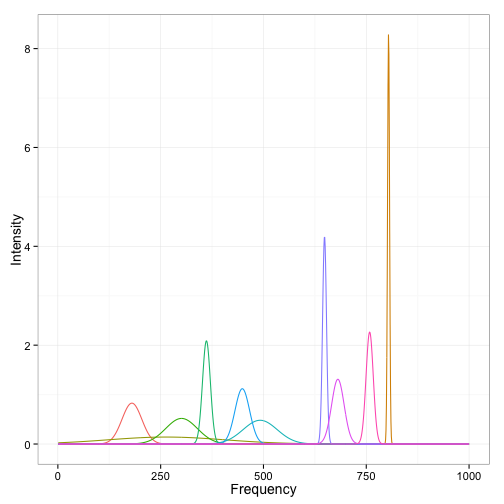We then set up our nonnegative least squares regression problem and utilize the MM algorithm described above to obtain a solution.

set.seed(12345)  n <- 100  p <- 3  X <- matrix(rexp(n * p, rate = 1), n, p)  b <- matrix(runif(p), p, 1)  y <- X %*% b + matrix(abs(rnorm(n)), n, 1)  sol <- nnls_mm(y, X, b) 

Next, we generate a small simulated de-mixing problem with noise added to a true mixture of 3 components.

## Setup mixture example  n <- 1000  p <- 10  nnm <- generate_nnm(n, p)   set.seed(12345)  X <- nnm\$X  b <- double(p)  nComponents <- 3  k <- sample(1:p, nComponents, replace = FALSE)  b[k] <- matrix(runif(nComponents), ncol = 1)  y <- X %*% b + 0.25 * matrix(abs(rnorm(n)), n, 1)   # Plot the mixture  plot_spect(n, y, X, b, nnm)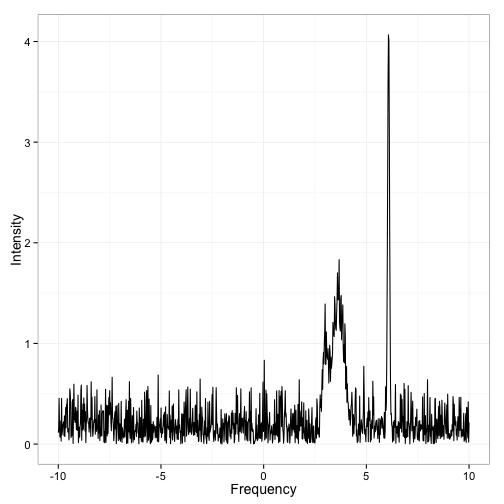The following plot shows the unadulterated mixture that we wish to de-mix.

# Plot the true signal  plot_nnm_truth(X, b, nnm)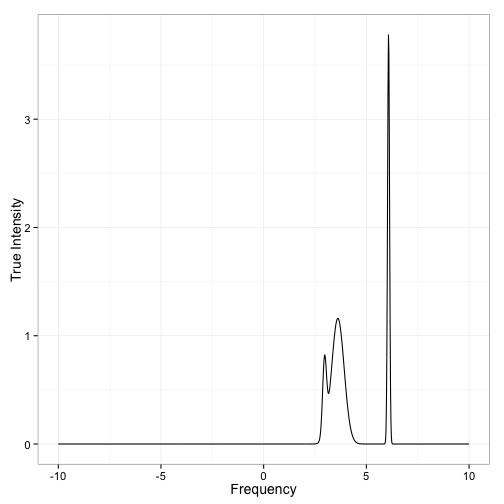The next few lines of R code show the resulting mixture estimated by nonnegative least squares.

# Obtain solution to mixture problem  nnm_sol <- nnls_mm(y, X, runif(p))   # Plot the reconstruction  plot_nnm_reconstruction(nnm, X, nnm_sol)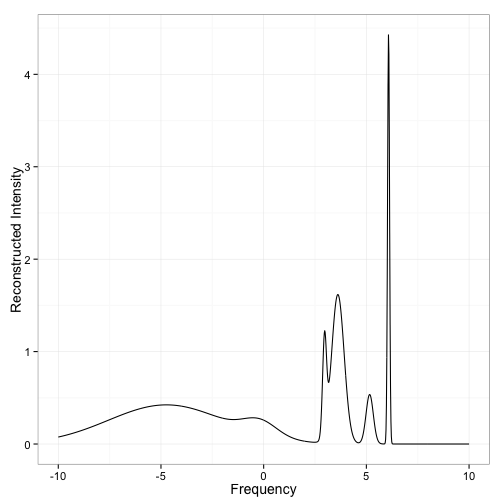The plot below shows the nonnegative regression components obtained using our algorithm.

# Plot the regression coefficients  plot_nnm_coef(nnm_sol)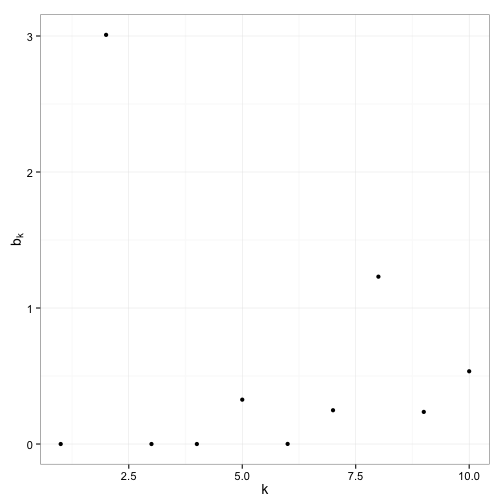The three largest components of the nonnegative least squares estimate coincide with the three true components in the simulated mixture. Nonetheless, the estimator also picked up several smaller false positives.

Before wrapping up with nonnegative least squares, we highlight the importance of verifying the monotonicity of our MM algorithm. The figure below depicts the decrease in the objective (or loss) function with each iteration in the algorithm after running it on the toy problem. As expected, the MM algorithm we utilize is monotonically decreasing. If this were not true, we would know immediately that something was amiss in either our code or our derivation of the algorithm.

set.seed(12345)  n <- 100  p <- 3  X <- matrix(rexp(n * p, rate = 1), n, p)  b <- matrix(runif(p), p, 1)  y <- X %*% b + matrix(abs(rnorm(n)), n, 1)  # Plot the objective  plot_nnm_obj(y, X, b)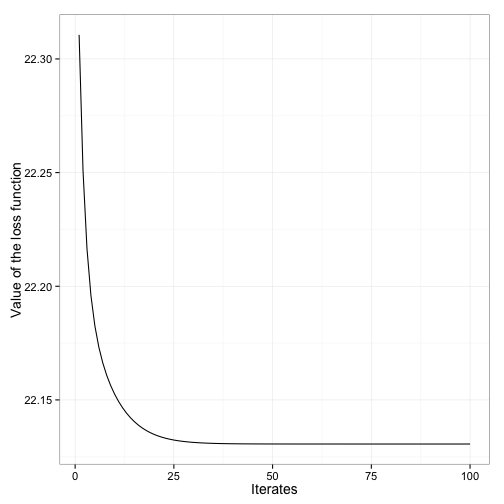## Conclusion

The ideas underlying the MM algorithm are extremely simple but when combined with a well-chosen inequality, they open the door to powerful and easily implementable algorithms for solving hard optimization problems. We readily admit that deriving an MM algorithm can be somewhat involved, as demonstrated in our examples. Nonetheless, the resulting algorithms are typically simple and easy to code. The matrix completion algorithm requires four simple steps, and the nonnegative least squares algorithm boils down to a multiplicative update that can be written in two lines of code. For both examples, the actual action part of the code rivals the amount of code needed to check for convergence in terms of brevity. Moreover, the monotonicity property provides a straightforward sanity check for the correctness of our code. We think most would agree with us that the time spent on messy calculus and algebra is worth it to avoid time spent debugging code.

In short, in the space of iterative methods, MM algorithms are relatively simple and can drastically cut down on code development time. While more sophisticated approaches might be faster in the end for any given problem, the MM algorithm allows us to rapidly evaluate the quality of our statistical models by minimizing the total time we spend developing ways to compute the estimators of parameters in those models. We have only skimmed the surface of the possible applications, and refer interested readers to Hunter and Lange for more examples.

## Footnote

1 Exploring the connection between the EM and MM algorithm is interesting in its own right and would require a separate article to do it justice. For the time being, however, we point the interested reader to several nice papers which take up this comparison. See 1, 2, 3, and 4. A line-by-line derivation which shows explicitly the minorization used in the EM algorithm is given in 1.

## Books & Journals

### Books#### Weight-of-Evidence for Forensic DNA Profiles, 2nd Edition#### Linear Algebra: Ideas and Applications, 4th EditionView all

### Journals#### Biometrical Journal#### WIREs Computational StatisticsView all